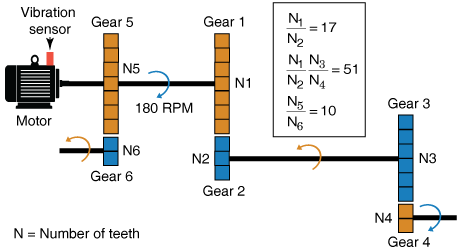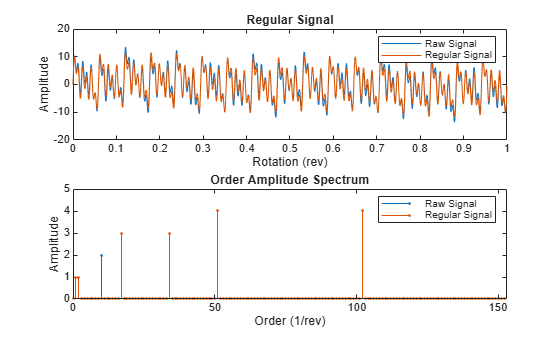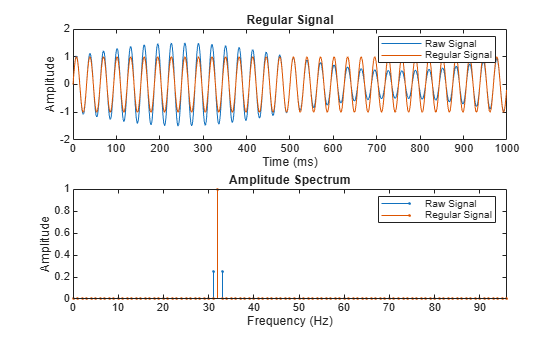Documentation

tsaregular

Regular signal of a time-synchronous averaged signal

Description

example

Y = tsaregular(X,fs,rpm,orderList) computes the regular signal Y of the time-synchronous averaged (TSA) signal vector X using sampling rate fs, the rotational speed rpm, and the orders to be retained orderList. Y is computed by retaining the primary frequency, the components in orderList, and their respective harmonics from X. You can use Y to further extract condition indicators of rotating machinery for predictive maintenance. For example, extracting the FM0 indicator from Y is useful in identifying major changes such as gear tooth breakage or heavy wear in a gear box.

example

Y = tsaregular(X,t,rpm,orderList) computes the regular signal Y of the TSA signal vector X with corresponding time values from t.

example

Y = tsaregular(XT,rpm,orderList) computes the regular signal Y of the TSA signal stored in the timetable XT. XT must contain a single numeric column variable.

example

[Y,S] = tsaregular(___) returns the amplitude spectrum S of the regular signal Y. S is the amplitude spectrum computed using the normalized fast Fourier transform (FFT) of Y.

example

___ = tsaregular(___,Name,Value) allows you to specify additional parameters using one or more name-value pair arguments. You can use this syntax with any of the previous input and output arguments.

example

tsaregular(___) with no output arguments plots the time-domain and frequency-domain plots of the raw and regular TSA signals.

Examples

collapse all

Consider a drivetrain with six gears driven by a motor that is fitted with a vibration sensor, as depicted in the figure below. Gear 1 on the motor shaft meshes with gear 2 with a gear ratio of 17:1. The final gear ratio, that is, the ratio between gears 1 and 2 and gears 3 and 4, is 51:1. Gear 5, also on the motor shaft, meshes with gear 6 with a gear ratio of 10:1. The motor is spinning at 180 RPM, and the sampling rate of the vibration sensor is 50 KHz. To retain the signal containing the meshing components of the gears 1 and 2, gears 3 and 4 and, the shaft rotation, specify their gear ratios of 17 and 51 in orderList. The signal components corresponding to the shaft rotation (order = 1) is always implicitly included in the computation.rpm = 180;
fs = 50e3;
t = (0:1/fs:(1/3)-1/fs)';                           % sample times
orderList = [17 51];
f = rpm/60*[1 orderList 10];

In practice, you would use measured data such as vibration signals obtained from an accelerometer. For this example, generate TSA signal X, which is the simulated data from the vibration sensor mounted on the motor.

X = sin(2*pi*f(1)*t) + sin(2*pi*2*f(1)*t) + ...     % motor shaft rotation and harmonic
3*sin(2*pi*f(2)*t) + 3*sin(2*pi*2*f(2)*t) + ... % gear mesh vibration and harmonic for gears 1 and 2
4*sin(2*pi*f(3)*t) + 4*sin(2*pi*2*f(3)*t) + ... % gear mesh vibration and harmonic for gears 3 and 4
2*sin(2*pi*10*f(1)*t);                          % gear mesh vibration for gears 5 and 6

Compute the regular signal of the TSA signal using the sample time, rpm, and the mesh orders to be retained.

Y = tsaregular(X,t,rpm,orderList);

The output Y is a vector containing everything except the gear mesh signal and harmonics for gears 5 and 6.

Visualize the regular signal, the raw TSA signal, and their amplitude spectrum on a plot.

tsaregular(X,fs,rpm,orderList)From the amplitude spectrum plot, observe the following components:

• The retained component at the 17th order and its harmonic at the 34th order

• The second retained component at the 51st order and its harmonic at the 102nd order

• The filtered mesh components for gears 5 and 6 at the 10th order

• The retained shaft component at the 1st and 2nd orders

• The amplitudes on the spectrum plot match the amplitudes of individual signals

In this example, sineWavePhaseMod.mat contains the data of a phase modulated sine wave. XT is a timetable with the sine wave data and rpm used is 60 RPM. The sine wave has a frequency of 32 Hz and to recover the unmodulated sine wave, use 32 as the orderList.

Load the data and the required variables.

ans=4×2 timetable
Time          Data
______________    _______

0 sec                   0
0.00097656 sec     0.2011
0.0019531 sec     0.39399
0.0029297 sec     0.57078

Note that the time values in XT are strictly increasing, equidistant, and finite.

Compute the regular signal and its amplitude spectrum. Set the value of 'Domain' to 'frequency' since the orders are in Hz.

[Y,S] = tsaregular(XT,rpm,orders,'Domain','frequency')
Y=1024×2 timetable
Time            Data
______________    __________

0 sec             -2.552e-15
0.00097656 sec       0.14928
0.0019531 sec        0.29283
0.0029297 sec        0.42512
0.0039062 sec        0.54108
0.0048828 sec        0.63624
0.0058594 sec        0.70695
0.0068359 sec        0.75049
0.0078125 sec         0.7652
0.0087891 sec        0.75049
0.0097656 sec        0.70695
0.010742 sec         0.63624
0.011719 sec         0.54108
0.012695 sec         0.42512
0.013672 sec         0.29283
0.014648 sec         0.14928
⋮

S = 1024×1 complex

0.0201 + 0.0000i
0.0971 - 0.1713i
0.2639 + 0.0945i
0.0025 + 0.0029i
-0.0008 - 0.0043i
-0.0009 - 0.0036i
-0.0006 - 0.0033i
-0.0019 + 0.0077i
0.0120 + 0.0035i
-0.0052 - 0.0008i
⋮

The output Y is a timetable that contains the regular signal, that is, the unmodulated sine wave, while S is a vector that contains the amplitude spectrum of the regular signal Y.

In this example, sineWaveAmpMod.mat contains the data of an amplitude modulated sine wave. X is a vector with the amplitude modulated sine wave data obtained at a shaft speed of 60 RPM. The unmodulated sine wave has a frequency of 32 Hz and amplitude of 1.0 units.

Load the data, and plot the regular signal of the amplitude modulated TSA signal X. To retain the unmodulated signal, specify the frequency of 32 Hz in orderList. Set the value of 'Domain' to 'frequency'.

tsaregular(X,t,rpm,orderList,'Domain','frequency');From the plot, observe the waveform and amplitude spectrum of the regular and raw signals, respectively. Observe that the regular signal contains the unmodulated sine wave with an amplitude of 1.0 units and frequency of 32 Hz.

Input Arguments

collapse all

Time-synchronous averaged (TSA) signal, specified as a vector. The time-synchronous averaged signal is computed from a long and relatively periodic raw signal through synchronization, resampling, and averaging. For more information on TSA signals, see tsa.

Time-synchronous averaging is a convenient method of background noise reduction in a spectrum of complex signals. It is effective in concentrating useful information that can be extracted from a time-domain signal for predictive maintenance. The synchronization typically requires a tachometer pulse signal in addition to the raw sensor data. The TSA signal depicts measurements at equally spaced angular positions over a single revolution of a shaft of interest.

Time synchronous averaged (TSA) signal, specified as a timetable. XT must contain a single numeric column variable corresponding to the TSA signal. Time values in XT must be strictly increasing, equidistant, and finite.

Sampling frequency of the TSA signal in Hertz, specified as a positive scalar.

Sample times of the TSA signal, specified as a positive scalar or a vector of positive values.

If t is:

• A positive scalar, it contains the time interval or duration between samples. You must specify t as a duration variable.

• A vector of positive values, it contains sample times corresponding to elements in X. The time values must be strictly increasing, equidistant, and finite. You can specify t as a double or duration variable.

Rotational speed of the shaft, specified as a positive scalar. tsaregular uses a bandwidth equal to the shaft speed and the value of 'NumSidebands' around the frequencies of interest to compute Y from the TSA signal. Specify rpm in revolutions per minute. The signal components corresponding to this frequency, that is, order = 1 are always retained.

Orders to be retained from the TSA signal, specified as a vector of positive integers. Select the orders and harmonics to be retained from the TSA signal by observing them on the amplitude spectrum plot. For instance, specify orderList as the known mesh orders in a gear train to retain the desired components and their harmonics. For more information, see Find and Visualize the Regular Signal of a Compound TSA Signal. Specify the units of orderList by selecting the appropriate value for 'Domain'.

Name-Value Pair Arguments

Specify optional comma-separated pairs of Name,Value arguments. Name is the argument name and Value is the corresponding value. Name must appear inside quotes. You can specify several name and value pair arguments in any order as Name1,Value1,...,NameN,ValueN.

Example: ...,'NumSidebands',2

Number of shaft and gear meshing frequency harmonics to be filtered, specified as the comma-separated pair consisting of 'NumHarmonics' and a positive integer. Modify 'NumHarmonics' if your TSA signal contains more than two known harmonics of components to be filtered.

Number of sidebands to be retained from the orderList frequencies and their harmonics, specified as the comma-separated pair consisting of 'NumSidebands' and a nonnegative integer. The width of sidebands is determined using 2*(rpm/60)*(NumSidebands+0.5). Modify 'NumSidebands' based on the number of sidebands to be retained from X as observed in the amplitude spectrum plot.

Number of shaft rotations in the TSA signal, specified as the comma-separated pair consisting of 'NumRotations' and a positive integer. Modify 'NumRotations' if your input X or XT contains data for more than one rotation of the driver gear shaft. The function uses 'NumRotations' to determine the number of rotations to be shown on the x-axis of the plot. The filtering results in Y are not affected by this value.

Units of the orderList values, specified as the comma-separated pair consisting of 'Domain' and one of the following:

• 'frequency', if the orders in orderList are specified as frequencies in units of Hertz.

• 'order', if the orders in orderList are specified as number of rotations relative to the value of rpm. For example, if the rotational speed of the driven gear is defined as a factor of the driver gear rpm, specify 'Domain' as 'order'. Also, choose 'order' if you are comparing data obtained from machines operating at different speeds.

Output Arguments

collapse all

Regular signal of the TSA signal, returned as:

• A vector, when the TSA signal is specified as a vector X.

• A timetable, when the TSA signal is specified as a timetable XT.

Y is computed by retaining the primary frequency, the components in orderList, the first-order sidebands in 'NumSidebands', and their respective harmonics from X. You can use Y to further extract condition indicators of rotating machinery for predictive maintenance. For example, extracting the FM0 indicator from Y is useful in identifying major changes such as gear tooth breakage or heavy wear in a gear box. For more information on how Y is computed, see Algorithms.

Amplitude spectrum of the regular signal, returned as a vector. S is the normalized fast Fourier transform of the signal Y. S is the same length as the input TSA signal X. For more information on how S is computed, see Algorithms.

Algorithms

Regular Signal

The regular signal is computed from the TSA signal by retaining the following from the signal spectrum:

• Shaft frequency and its harmonics

• Gear meshing frequencies and their harmonics

• Optionally, the sidebands specified in 'NumSidebands' at the gear meshing frequencies and their harmonics

tsaregular uses a bandwidth equal to the shaft speed times the value of 'NumSidebands', around the frequencies of interest, to compute Y from the TSA signal. The regular signal is related to the residual signal through the equation . If the first-order sidebands are retained in the regular signal, then, .

Amplitude Spectrum

The amplitude spectrum of the regular signal is computed as follows,

Here, Y is the regular signal.

 McFadden, P.D. "Examination of a Technique for the Early Detection of Failure in Gears by Signal Processing of the Time Domain Average of the Meshing Vibration." Aero Propulsion Technical Memorandum 434. Melbourne, Australia: Aeronautical Research Laboratories, Apr. 1986.

 Večeř, P., Marcel Kreidl, and R. Šmíd. "Condition Indicators for Gearbox Monitoring Systems." Acta Polytechnica 45.6 (2005), pages 35-43.

 Zakrajsek, J. J., Townsend, D. P., and Decker, H. J. "An Analysis of Gear Fault Detection Methods as Applied to Pitting Fatigue Failure Data." Technical Memorandum 105950. NASA, Apr. 1993.

 Zakrajsek, James J. "An investigation of gear mesh failure prediction techniques." National Aeronautics and Space Administration Cleveland OH Lewis Research Center, 1989. No. NASA-E-5049.